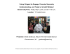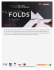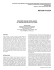# Origami (from ori meaning folding, and kami meaning paper

## Transcription

Origami (from ori meaning folding, and kami meaning paper
```Use ORIGAMI to introduce geometry and algebra ideas.
Origami
(from ori meaning folding, and kami meaning paper)
Origami is the Japanese art of paper folding.
It started in the 17th century AD and was popularized in the
mid-1900s.
In 1930 Akira Yoshizawa, a Japanese origami artist/writer,
comes up with a way of illustrating the steps. This
revitalized origami throughout the world.
In origami the goal is to turn a flat piece of paper into a three
dimensional sculpture.
Cutting and gluing are not acceptable.
Traditionally a square sheet of paper is used
(but it is okay to break the rule!)
Famous Names in Origami
Akira Yoshizawa
Japanese Origami Artist/Writer
(1911-2005)
Tomoko Fuse
Japanese Origami Artist/Writer
(1951-)
Robert J. Lang
American Physicist/Mathematician/Origami Artist
(1950-)
Erik Demaine
Artist
(1981-)
Can origami save life?
Zhong You and Kaori Kuribayashi
Department of Engineering Science
University of Oxford
Oxford, OX1 3PJ
U. K.
One of the goals of the contemporary reform movement is to make many of
the abstract ideas of mathematics concrete (whenever possible).
Origami helps students engage in spatial visualization, and communicate
better.
Origami star.
PART I:
Making a STAR
Directions:
It takes 8 pieces of paper to
complete the STAR.
Stage 1
Fold along dotted lines.
There are three folds shown
here.
Stage 2
Fold two corners down. Use the
midpoints of the sides as a guide.
Stage 3
Turn the paper over so that it looks
like this. Then press down on point A
as you fold segments BC and DE
together. The result is a
parallelogram.
C
B
D
A
E
Stage 4
Once you have folded 8 parallelograms, connect them by placing one inside the fold of
another. To make the connection, fold the points of one parallelogram.
Finally, slide the opposite sides to form the star.
PART II:
VOCABULARY:
DISTANCE
MIDPOINT
DIAGONAL
INTERSECT
ALTITUDE
CONGRUENT
TRIANGLES
LINE OF SYMMETRY
SLOPE
PERPENDICULAR
PARALLELOGRAM
OPPOSITE SIDES
OPPOSITE ANGLES
OCTAGON
POLYGON
Discussion questions:
Stage 1
Describe symmetry with stage 1? Think about symmetry with respect to a point
or a side. Use rotation, reflection, or translation to describe a transformation that
carries part of the figure onto another.
(G-CO-5)
Given a geometric figure and a rotation, reflection, or translation, draw the transformed
figure using, e.g., graph paper, tracing paper, or geometry software. Specify a sequence
of transformations that will carry a given figure onto another.
Stage 2
How do we know that the dotted lines connect midpoints in the figure?
Stage 3
How can the following formulas help to show that the figure is a parallelogram?
1) Distance
2) Midpoint
3) Slope
Use graph paper and the formulas above to show that the quadrilateral is a
parallelogram
Part III:
Area, Pythagorean Theorem, and Special Right Triangles (a little algebra)
Look at stage 1.
Find the area of the square in as many ways as possible.
A.
B.
C.
D.
E.
F.
length x width
half x diagonal1 x diagonal2
4 triangles
Half x apothem x perimeter
8 triangles
2 triangles
Give a general formula for the area of a square that uses the following information only:
1.
2.
3.
4.
only the lengths of the sides
only the lengths of the diagonals
only the apothem
Explain how 1 and 4 are related.
Critical questions:
What algebra skills are used?
What vocabulary words are used?
What special triangles are used?
For each part of the exercise above, make a list of CCSS that apply.
Mathematics and Origami
Arsalan Wares
Department of Math and CS
Valdosta State University
Valdosta, GA
The Optimal Origami Box
Math in Action
Feb. 22, 2007
Shelly Smith
Grand Valley State University
http://faculty.gvsu.edu/smithshe
A hands-on activity where students fold origami boxes with varying heights to explore
modeling
and optimization. You can download this activity from my website and modify it for use
in your
class.
Adapted from Unfolding Mathematics with Origami Boxes, by Arnold Tubis and Crystal
Mills.
Materials:
• Folding instructions: Wikipedia
• Square origami (scrapbooking) paper
• Rulers
• graphing calculators
Magazine Box
Magazine box crease pattern.
w/2
h
l/2
L
W
L  l  2h  2whem
W  w  2h
Question 1:
What size paper is required for folding a Magazine Box of
length 4", width 3", height 2", and a hem width of 1"?
Question 2:
A Magazine Box with:
Length = width = twice the height is folded from an 8 ½” x 11"
sheet of paper.
Determine the hem width.
Some Mathematical Concepts and
Techniques Involved in Studies of the
Generalized Masu Designs
•
•
•
•
•
•
•
•
Algebraic Equations
Angles
Area and Volume
Arithmetic
Bisection (line, angle)
Calculator Math
Comparison of theoretical and actual
measure or box parameters
Congruence (verified by folding)
•
•
•
•
•
•
•
•
•
Fractions and ratios
Graphical analysis
Maxima/minima of box parameters
Percent error
Polygons (triangles, rectangles, . . . )
Pythagorean theorem
Rectangular solid
Spatial visualization
Symmetry
The Optimal Origami Box
In this activity, we will fold origami boxes with varying height, and determine which
height will give us a box with the largest possible volume.
Folding diagram from Wikipedia entry for Japanese masu
http://en.wikipedia.org/wiki/Masu_%28Japanese%29
The height will be half of the length of the base. To create a box with a different height,
modify steps 3 and 5 by making folded sides larger or smaller.
Folding Boxes and Using Data to Create a Model
1.
Using your square sheet of paper, fold a box following the instructions given.
Note that since our paper is square, the base of the box is also square. (What does
this tell you about the length and width of the box?) Measure your box and
2.
Suppose we decrease the height of the box. What do you predict would happen to
the length and width of the box? What about the volume of the box?
3.
Suppose we increase the height of the box. What do you predict would happen to
the length and width of the box? What about the volume of the box?
4.
In your group, fold boxes different heights, and calculate the volume of each to
fill in the following table. (Two rows are left blank for data for boxes with zero
volume.)
Height
1
1.5
2.125
2.5
Length
Width
Volume
5.
Enter the data from your table into your calculator to create a scatter plot of the
data and sketch it below.
6.
What type of model do you choose to approximate your scatter plot? Write the
function below and graph your model on the same set of axes with the scatter plot.
Does it seem like a reasonable approximation of your data? If not, can you choose
a more accurate model?
7.
Use your model from question 6 to determine the height should we use to
maximize the volume of an origami box. What is the maximum possible volume
Creating a Theoretical Model
8.
What is the general formula for the volume of a box with a rectangular base? This
formula has too many variables, but already we can eliminate one of them
because our box has a square base. What is the modified formula?
9.
Unfold the boxes that you made so that we can see the creases. They should look
similar to the diagram below. Trace the creases that outline the base of your box
and the creases that outline the sides. What is the length of the dashed diagonal
10.
Identify segments of the diagonal lines on the crease diagram that can be used to
measure the length and height of the box. Use these segments to find an equation
that relates the length of the diagonal to the height, H, and length, L, of the box.
(You may want to include the equation ____L+____H=____.) How can we use
this equation to eliminate another variable from the volume formula?
11.
We now have a formula for the volume of an origami box as a function of its
height.
(For algebra)
Graph the volume function on your calculator and find the optimal height and
maximum volume of an origami box. How does this compare to your model from
the data?
(For calculus)
a) Differentiate the volume function and find its critical points.
b) Which critical point is the optimal height of your origami box?
c) What is the maximum volume for your origami box?
d) How do these results compare to your results in question 7?
What if we change the size of the paper that we use?
12.
If we change the size of the paper, how would that change the equation that you
found in question 10 and the resulting volume function?
13.
(For algebra)
Find the optimal height and maximum volume of an origami box folded from a
12” x 12” square sheet of paper.
(For calculus)
Find the optimal height and maximum volume of an origami box folded from a
square sheet of paper with sides S inches long. Remember that S is a constant
when you are creating and differentiating your volume function, and the input
variable is H.
References
 H. Gardner, Multiple Intelligences: New Horizons, Basic Books, New York, NY,
2006.
 H. Gardner, Intelligences Reframed, Basic Books, New York, NY, 1999.
 Ubiratan D’Ambrosio, General Remarks on Ethnomathematics, ZDM (Zentralblatt
für Didaktik der Mathematik). 33-3 (2001), pp. 67–69.
```

### PDF Flyer - Friends of the Fort Lauderdale Libraries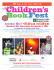### “FLorigami” Turtle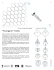### How to Make an Origami Heart in 14 Steps (with...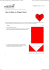### Modern Origami.key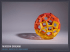### Symposium on Origami-Based Engineering Design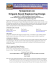### Topological Origami Models of Non-Convex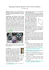### Boxin the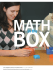### may the folds be with you!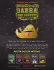### Origami constructions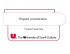### win... - Viking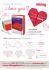### Here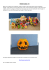### How to make Origami Flowers - Zikra Toure E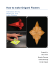### Elementary algebra for Origami: The trisection problem revisited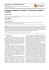### Around The World in 30 Sonobe Units: An Origami Globe of the Earth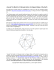### PaperCraftCentral.net - All About PaperCrafts and Origami### Contents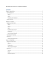### this Release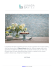### How to Make Origami Wishing Stars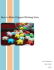### May 2013 Dear Middle School Families, Trying to choose a quote to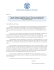### Using Origami to Engage, Promote Geometryаа Understanding, and